# 【C++】C++入门

### 文章目录

• 1.C++的由来
• 2.C++关键字(C++98)
• 2.1. 命名空间
• 2.1.1.C语言的命名冲突
• 2.1.2.命名空间定义
• 2.2.命名空间使用
• 3.C++输入&输出
• 4.缺省参数
• 4.1.缺省参数概念
• 4.2.缺省参数分类
• 5.函数重载
• 5.1.函数重载概念
• 5.2.函数重载的原理 (重要)
• 6.内联函数
• 6.1.内联函数的概念
• 6.2.内联函数的特性
• 7.auto关键字(C++11)
• 7.1.类型别名思考
• 7.2.auto 的概念
• 7.3.auto 的使用细则
• 7.4.auto不能推导的场景
• 8.基于范围的 for 循环 (C++11)
• 8.1.范围 for 的用法
• 8.2.范围 for 的使用条件
• 9.指针空值 nullptr (C++11)

# 1.C++的由来

C语言是结构化和模块化的语言，适合处理较小规模的程序。对于复杂的问题，规模较大的程序，需要高度的抽象和建模时，C语言则不合适。为了解决软件危机， 20世纪80年代， 计算机界提出了OOP(object oriented programming：面向对象)思想，支持面向对象的程序设计语言应运而生。 1982年，贝尔实验室的Bjarne Stroustrup博士在C语言的基础上引入并扩充了面向对象的概念，发明了一种新的程序语言。为了表达该语言与C语言的渊源关系，命名为C++，Bjarne Stroustrup因此也被尊称为C++之父。因此C++是基于C语言而产生的，它既可以进行C语言的过程化程序设计，又可以进行以抽象数据类型为特点的基于对象的程序设计，还可以进行面向对象程序设计。C++是在C的基础之上，容纳进去了面向对象编程思想，并增加了许多有用的库，以及编程范式等。熟悉C语言之后，对C++学习有一定的帮助。

C++之父 :本贾尼·斯特劳斯特卢普# 2.C++关键字(C++98)

C++总计63个关键字，C语言32个关键字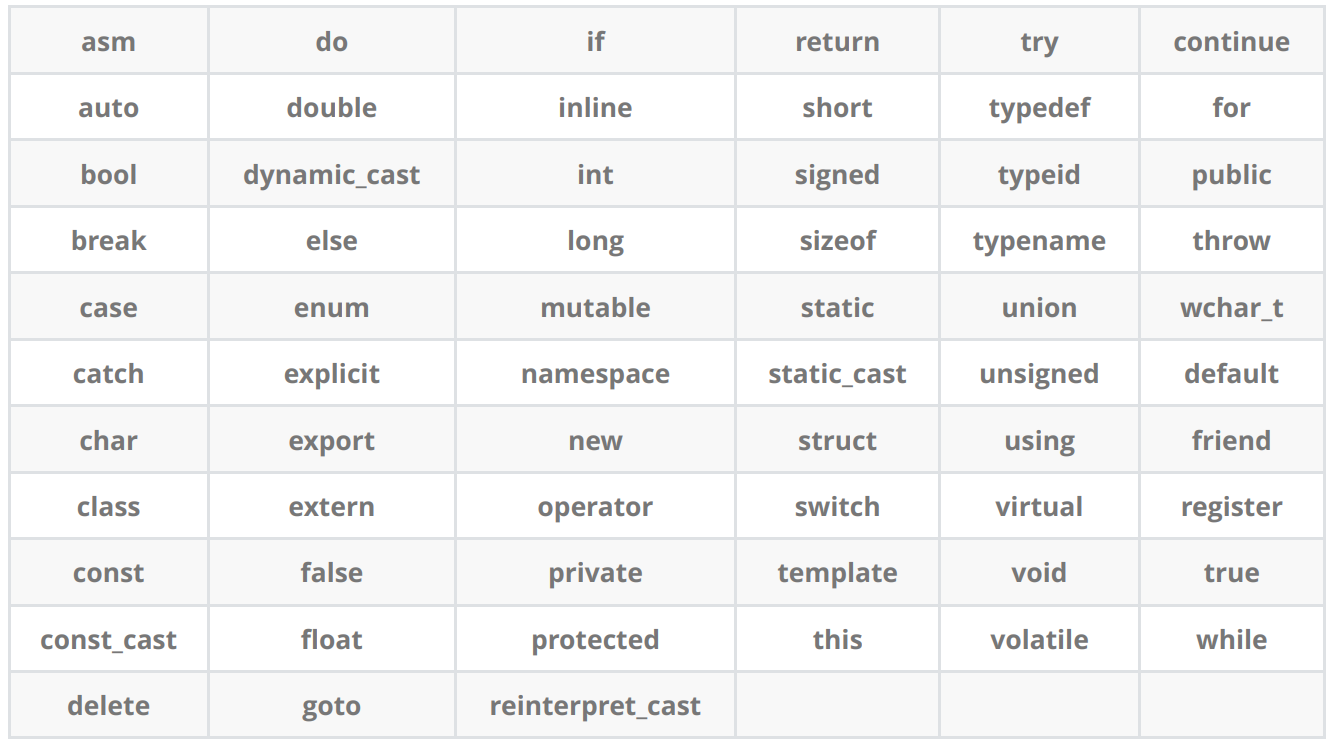## 2.1. 命名空间

``````#include<iostream>
``````

``````using namespace std;
``````

namespace就是我们要接触C++的第一个关键字，它就是**命名空间**。

• 命名空间的作用：

### 2.1.1.C语言的命名冲突

``````#include <stdio.h>
#include <stdlib.h>
int rand = 10;

int main()
{
printf("%d\n", rand);
return 0;
}
``````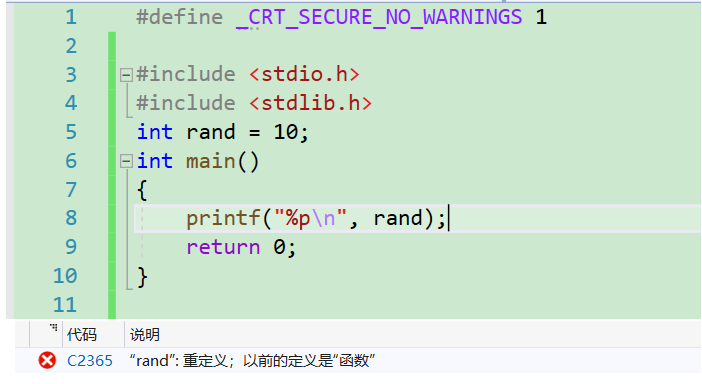<stdlib.h>。这里很明显发生命名冲突了，我们定义的全局变量rand和库里的rand函数冲突。想要解决此问题也非常简单，修改变量名就可以了，确实可以，但并不是长久之计，万一你不小心在程序中定义了多个与库函数冲突的函数，难道你要一个一个修改吗，这就充分体现了C语言的命名冲突。

C语言没办法解决类似这样的命名冲突问题，所以C++提出了namespace来解决。

### 2.1.2.命名空间定义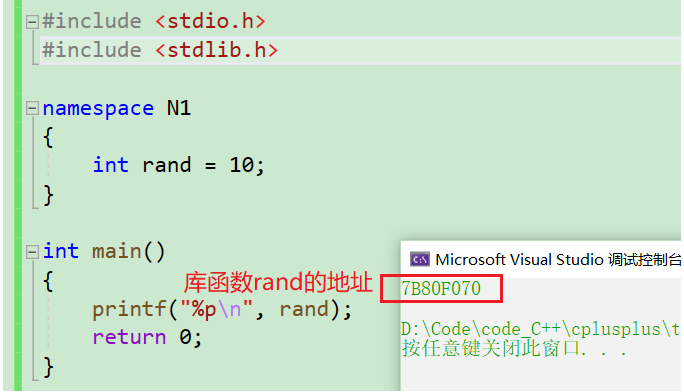1.命名空间可以定义变量，函数，类型

``````//命名空间不影响变量的生命周期，只是限定域，编译查找规则
namespace bit //bit为命名空间的名称
{
int rand = 11;//变量
int x = 0;
int Add(int left, int right) //函数
{
return left + right;
}
struct Node // 类型
{
struct Node* cur;
int val;
};
}
``````

2.命名空间可以嵌套

``````namespace N1
{
int a;
int b;
{
return left + right;
}
namespace N2
{
int c;
int d;
int Sub(int left, int right)
{
return left - right;
}
}
}
``````

3.3. 同一个工程中允许存在多个相同名称的命名空间,编译器最后会合成同一个命名空间中。
ps：一个工程中的test.h和上面test.cpp中两个N1会被合并成一个。

``````//test.h
namespace N1
{
int Mul(int left, int right)
{
return left * right;
}
}
``````

## 2.2.命名空间使用

``````int a = 0; // 全局变量
int main()
{
int a = 1; //局部变量
printf("%d\n", a);// 1
return 0;
}
``````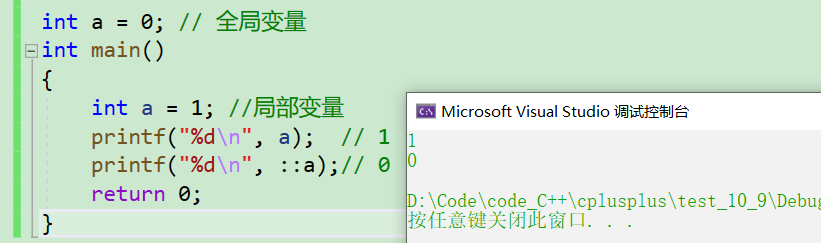``````namespace bit
{
int rand = 0;
char c = 'a';
}
``````

1.使用作用域限定符::

``````int main()
{
printf("%d\n", bit::rand);
printf("%d\n",bit::c);
return 0;
}
``````

``````namespace bit //bit为命名空间的名称
{
int rand = 11;//变量
int x = 0;
int Add(int left, int right) //函数
{
return left + right;
}
struct Node // 类型
{
struct Node* cur;
int val;
};
}
//避免命名冲突
namespace byte
{
struct Node
{
struct Node* cur;
struct Node* prev;
int val;
};
}
``````

``````int main()
{
struct bit::Node node;
struct byte::Node dnode;
}
``````

``````namespace N1
{
int a;
int b;
{
return left + right;
}
namespace N2
{
int c;
int d;
int Sub(int left, int right)
{
return left - right;
}
}
}
``````

``````main()
{
N2::c = 2; //false
N1::N2::c = 2;//true
printf("%d\n", N1::N2::c);
printf("%d\n",N1::N2::Sub(3, 1));
return 0;
}
``````

2.使用using namespace 命名空间全部展开

``````using namespace N1;
``````

``````int main()
{
N2::c = 4;
printf("%d\n", N2::c);
printf("%d\n", N2::Sub(3, 1));
}
``````

``````using namespace N1;
using namespace N2;
int main()
{
c = 6;
printf("%d\n", Sub(3, 1));
}
``````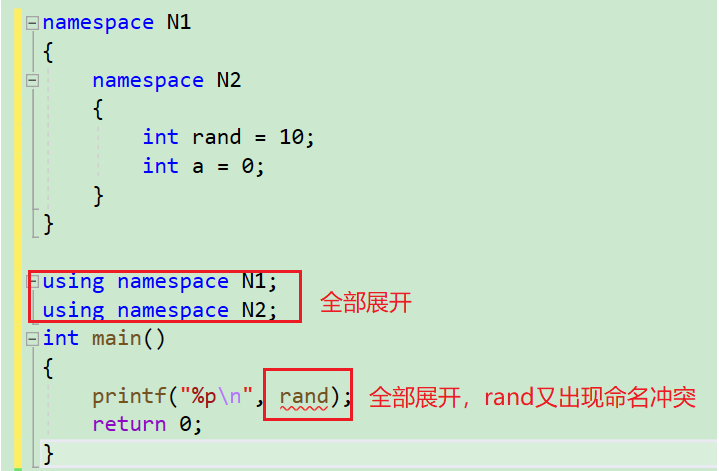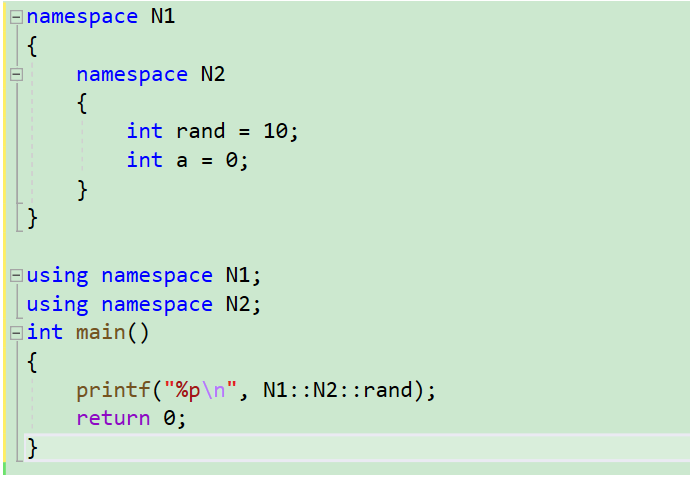3.使用using将命名空间部分展开

``````namespace N1
{
int rand = 10;
int a = 0;
}
using N1::a;
int main()
{
a++;
printf("%d\n", a);
N1::rand++;
printf("%d\n", N1::rand);
}
``````

``````#include <iostream>

using namespace std; // std是封装C++库的命名空间
int main()
{
cout << "hello world!" << endl;//打印"hello world!"
return 0;
}
``````

``````using namespace std;
``````

``````#include <iostream>

int main()
{
std::cout << "hello world!" << std::endl;//打印"hello world!"
return 0;
}
``````

``````#include <iostream>
using std::cout;
int main()
{
cout << "hello world!" << std::endl;//打印"hello world!"
}
``````

std命名空间的使用惯例：

std是C++标准库的命名空间，如何展开std使用更合理呢？

1. 在日常练习中，建议直接using namespace std即可，这样就很方便。

2. using namespace std展开，标准库就全部暴露出来了，如果我们定义跟库重名的类型/对象/函数，就存在冲突问题。该问题在日常练习中很少出现，但是项目开发中代码较多、规模大，就很容易出现。所以建议在项目开发中使用，像std::cout这样使用时指定命名空间 +using std::cout展开常用的库对象/类型等方式。这就充分运用到了命名空间，希望大家能活学活用。

# 3.C++输入&输出

``````#include <iostream>

using namespace std; // std是封装C++库的命名空间
int main()
{
cout << "hello world!" << endl;//打印"hello world!"
return 0;
}
``````

1. 使用cout标准输出对象(控制台)cin标准输入对象(键盘)时，必须包含< iostream >头文件 以及按命名空间使用方法使用std。

2. cout和cin是全局的流对象，endl是特殊的C++符号，表示换行输出，他们都包含在包含< iostream >头文件中。

3. <<是流插入运算符，>>是流提取运算符

4. 使用C++输入输出更方便，不需要像printf/scanf输入输出时那样，需要手动控制格式。 C++的输入输出可以自动识别变量类型

5. 实际上cout和cin分别是ostream和istrea 类型的对象，>>和<<也涉及运算符重载等知识， 这些知识我们我们后续才会学习，所以我们这里只是简单学习他们的使用。后面我们再深入学习。

6.endl是换行符，等价于C语言的’\n’。

# 4.缺省参数

## 4.1.缺省参数概念

``````void Func(int a = 0)
{
cout<<a<<endl;
}

int main()
{
Func(); // 没有传参时，使用参的默认值
Func(10); // 传参时，使用指定的实参
return 0;
}
``````

## 4.2.缺省参数分类

``````void Func(int a = 10, int b = 20, int c = 30)
{
cout << "a = " << a << endl;
cout << "b = " << b << endl;
cout << "c = " << c << endl;
}
``````

``````int main()
{
Func();
Func(1);
Func(1, 2);
Func(1, 2, 3);
//Func(1, , 3);//false
}
``````

``````//半缺省/部分缺省 -- 只能从左往右缺省
void Fun3(int a, int b = 20, int c = 30)
{
cout << " a = " << a;
cout << " b = " << b;
cout << " c = " << c << endl;
}
``````

``````int main()
{
Func3(1);
Func3(1, 2);
Func3(1, 2, 3);
return 0;
}
``````

1. 半缺省参数必须从右往左依次来给出，不能间隔着给

``````//错误示范
void Fun3(int a = 10, int b = 20, int c)
{
cout << " a = " << a;
cout << " b = " << b;
cout << " c = " << c << endl;
}
``````

2. 缺省参数不能在函数声明和定义中同时出现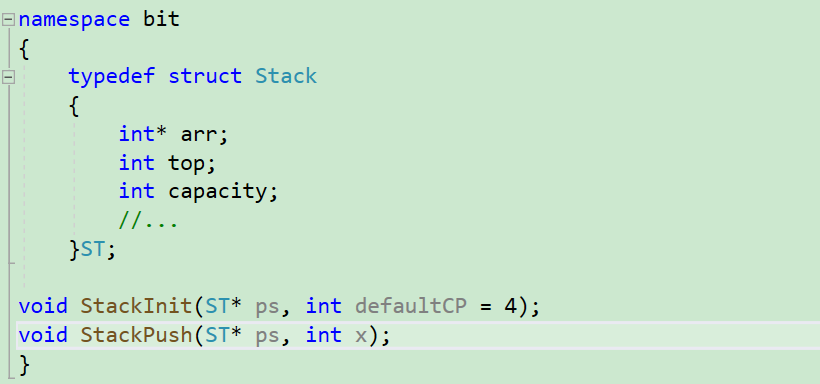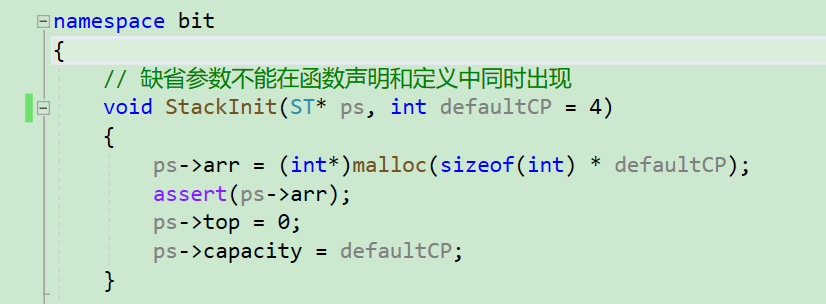1.缺省参数不能在定义和声明中同时出现，防止出现不同的赋值导致错误

2. 缺省值必须是常量或者全局变量。

3. C语言不支持（编译器不支持）

# 5.函数重载

## 5.1.函数重载概念

• 参数类型不同
``````#include<iostream>
using namespace std;

//参数类型不同构成函数重载
{
cout << "int Add(int left, int right)" << endl;
return left + right;
}
{
cout << "double Add(double left, double right)" << endl;
return left + right;
}
``````
• 参数个数不同
``````//参数个数不同构成函数重载
void f()
{
cout << "f()" << endl;
}
void f(int a)

{
cout << "f(int a)" << endl;
}
``````
• 顺序不同
``````//参数类型顺序不同构成函数重载
void f(int a, char b)
{
cout << "f(int a,char b)" << endl;
}
void f(char b, int a)
{
cout << "f(char b, int a)" << endl;
}
``````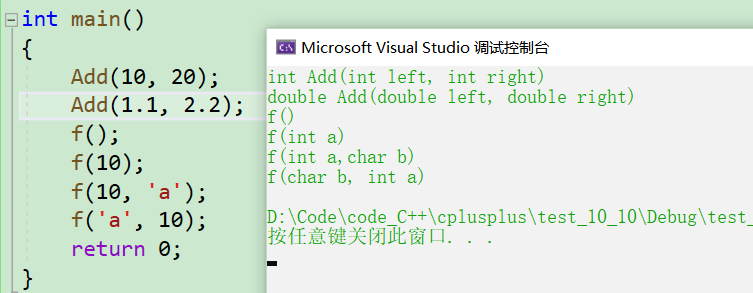## 5.2.函数重载的原理 (重要)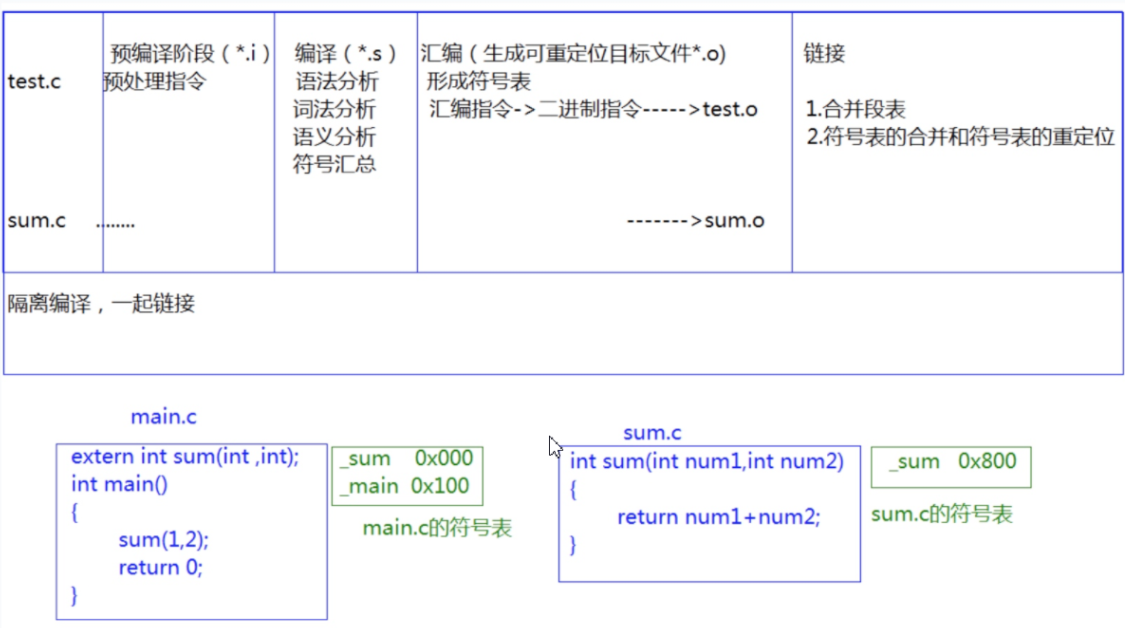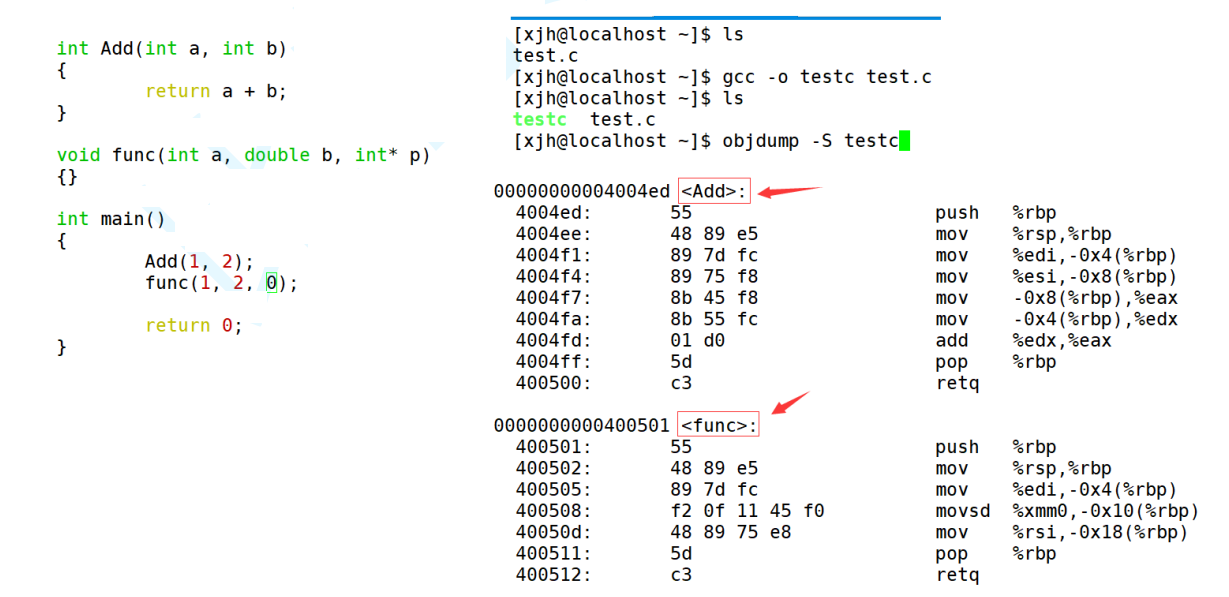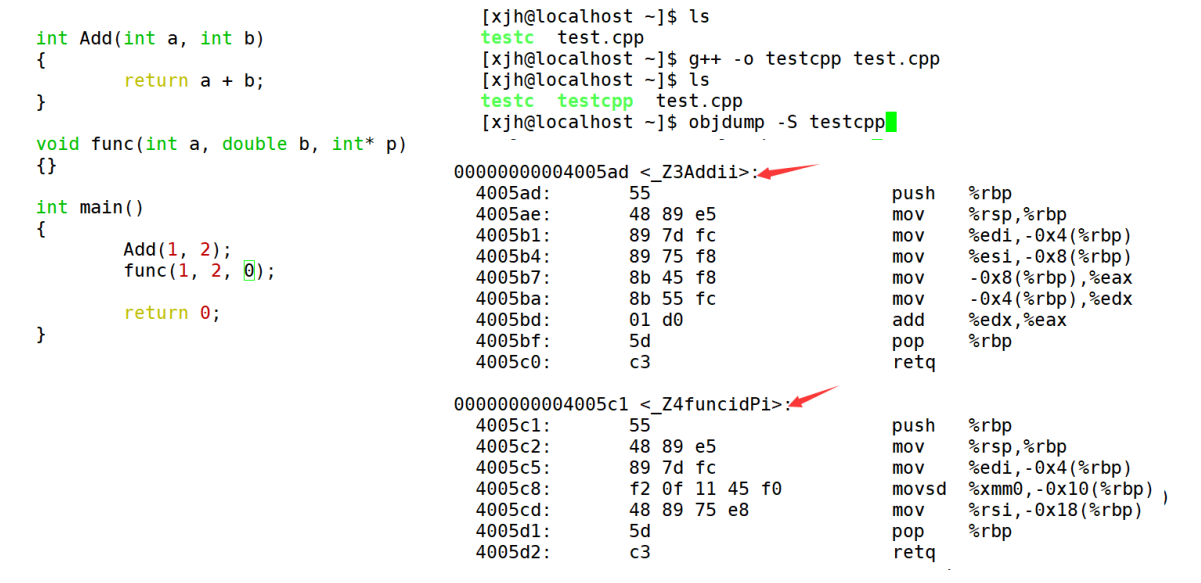# 6.内联函数

## 6.1.内联函数的概念

``````void Swap(int* x, int* y)
{
int tmp = *x;
*x = *y;
*y = tmp;
}
``````

``````#define SWAP(x, y) {int tmp = x; x = y; y = tmp;}
``````
• 既然用宏就可以解决，C++为何引出inline?

1. 解决宏函数比较难写，容易写错的问题
2. 解决宏不支持调试
3. 不支持类型安全的检查等问题
• 内联函数（inline）如何使用？

``````inline int Add(int x, int y)
{
return x + y;
}
``````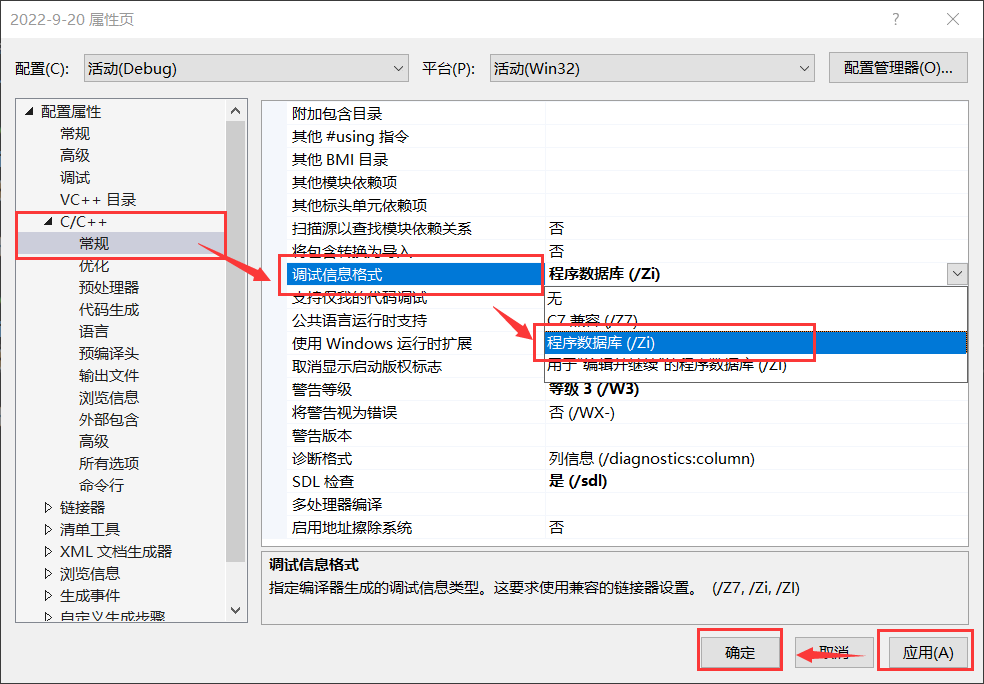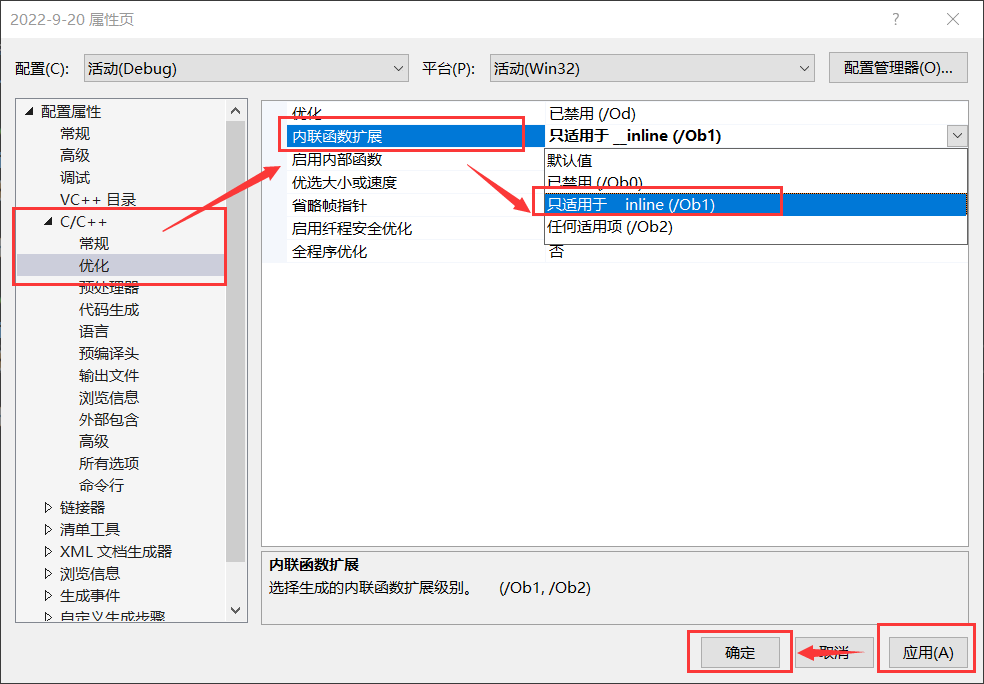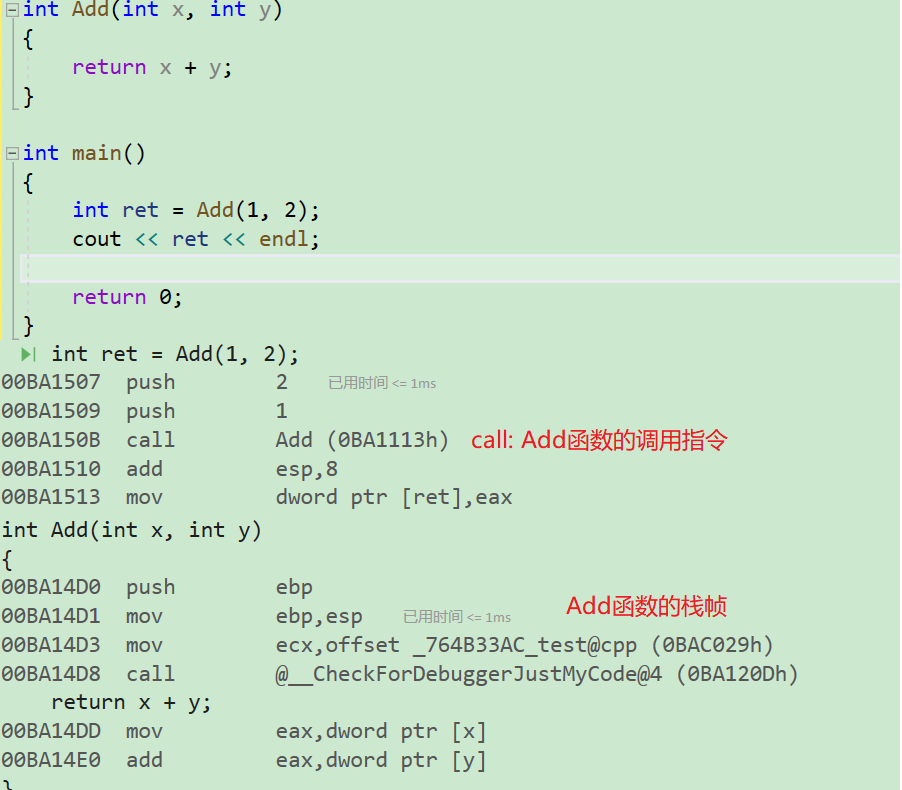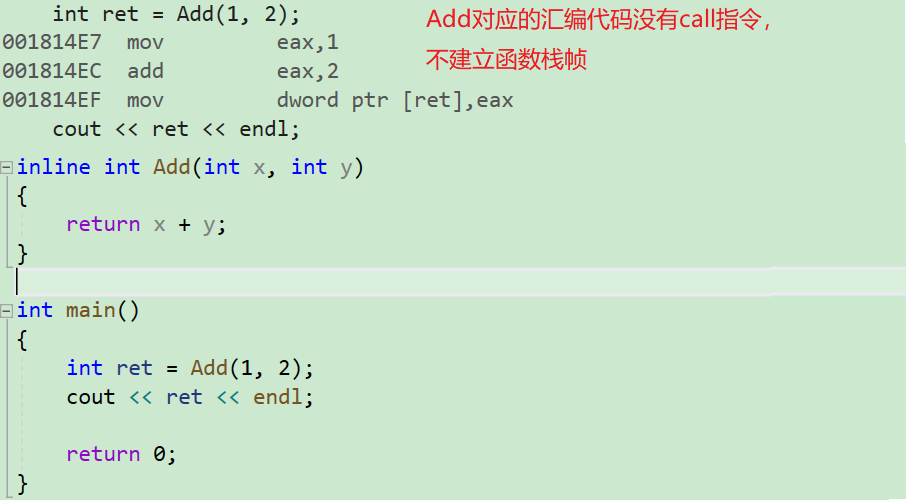inline的好处如下：

1. debug支持调试
2. 不易写错，就是普通函数的写法

## 6.2.内联函数的特性

inline 对于编译器而言只是一个建议 (类似于C语言的 register 关键字)，不同编译器关于 inline 的实现机制可能不同，一般建议将具有如下特点的函数采用 inline 修饰：

• 函数规模较小 (即函数不是很长，具体没有准确的说法，取决于编译器内部实现)；
• 不是递归
• 频繁调用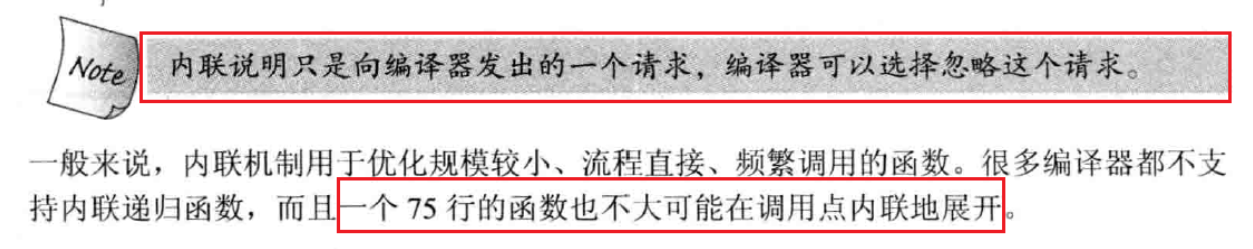``````//把Add的内部逻辑复杂化
inline int Add(int x, int y)
{
int ret = x + y;
ret += x + y;
ret = x + y;
ret /= x + y;
ret = x + y;
ret = x + y;
ret *= x + y;
ret = x + y;
ret = x + y;
ret -= x + y;
ret = x + y;
ret += x + y;
return ret;
}
``````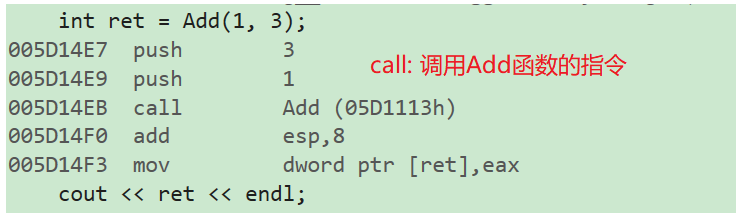inline 是一种以空间换时间的做法，如果编译器将函数当成内联函数处理，在编译阶段会用函数体替换函数调用；这样做的优势是减少了栈帧建立的开销，提高了程序运行效率；缺陷是可能会使目标文件变大。

inline 不建议声明和定义分离，分离会导致链接错误，具体原因如下：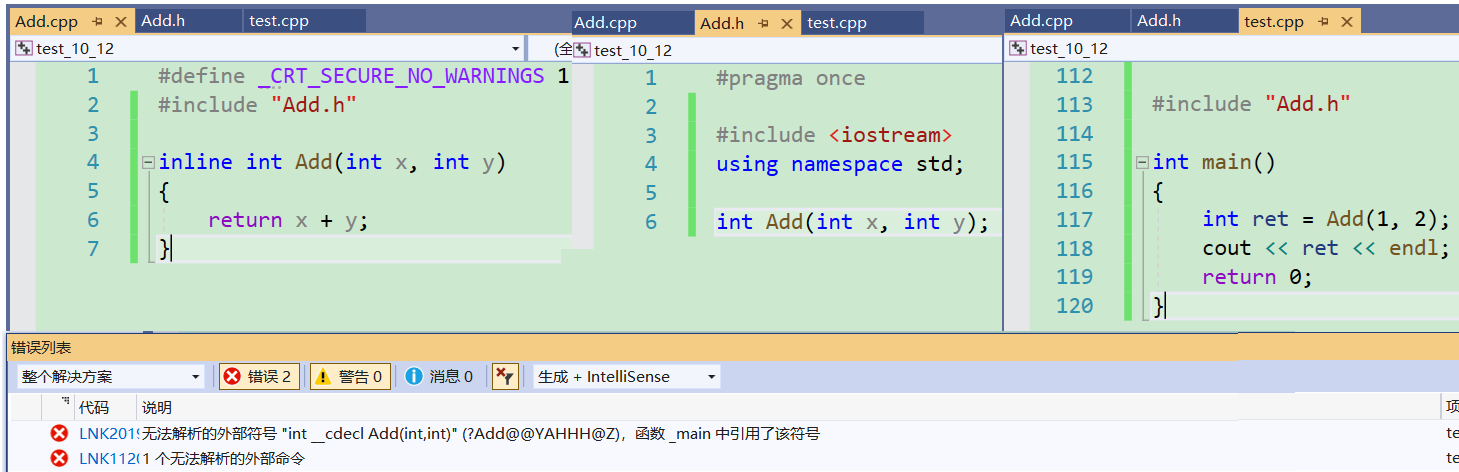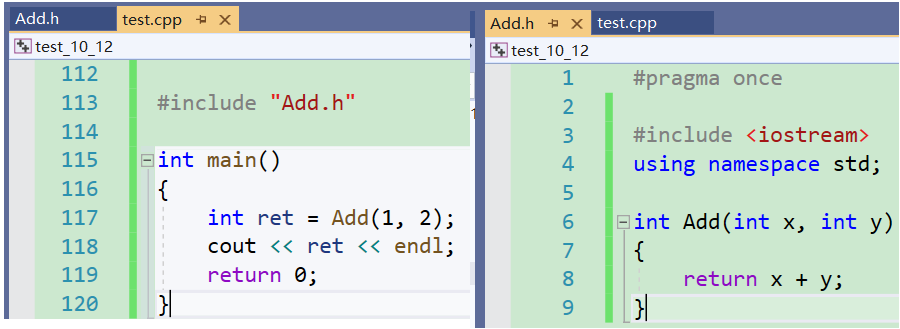【经典面试题】

• 问题1：宏的优缺点？

1. 增强代码的复用性
2. 提高性能

1. 不方便调试宏（因为预编译阶段进行了替换）
2. 导致代码可读性差，可维护性差，容易误用
3. 没有类型安全的检查
• 问题2：C++有哪些技术代替宏？
1. 常量定义，换用const，enum
2. 函数定义，换用内联函数

# 7.auto关键字(C++11)

## 7.1.类型别名思考

• 类型难于拼写；

• 含义不明确导致容易出错；

``````#include <string>
#include <map>
int main()
{
std::map<std::string, std::string> m{ { "apple", "苹果" }, { "orange","橙子" },{"pear","梨"} };
std::map<std::string, std::string>::iterator it = m.begin();
while (it != m.end())
{
//....
}
return 0;
}
``````

std::map::iterator 是一个类型，但是该类型太长了，特别容易写错；可能聪明的同学可能已经想到：我们可以通过 typedef 给类型取别名，比如：

``````#include <string>
#include <map>
typedef std::map<std::string, std::string> Map;
int main()
{
Map m{ { "apple", "苹果" },{ "orange", "橙子" }, {"pear","梨"} };
{
//....
}
return 0;
}
``````

``````typedef char* pc;
int main()
{
//能否编译成功？
const pc p1;
cosnt pc* p2;
return 0;
}
``````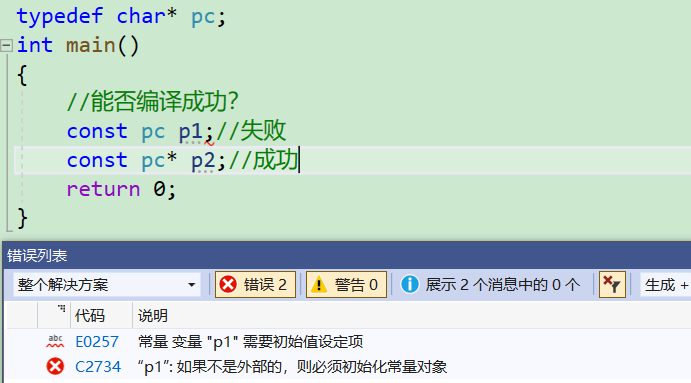## 7.2.auto 的概念

``````int a = 10;
auto b = a;
auto c = 'a';
``````

• 补充：这里补充一个知识点：typeid().name：它是专门用来输出一个变量的类型，返回的是一个字符串

``````int TestAuto()
{
return 10;
}
int main()
{
int a = 10;
auto b = a;
auto c = 'a';
auto d = TestAuto();
cout << typeid(b).name() << endl;
cout << typeid(c).name() << endl;
cout << typeid(d).name() << endl;
//auto e; 无法通过编译，使用auto定义变量时必须对其进行初始化
return 0;
}
``````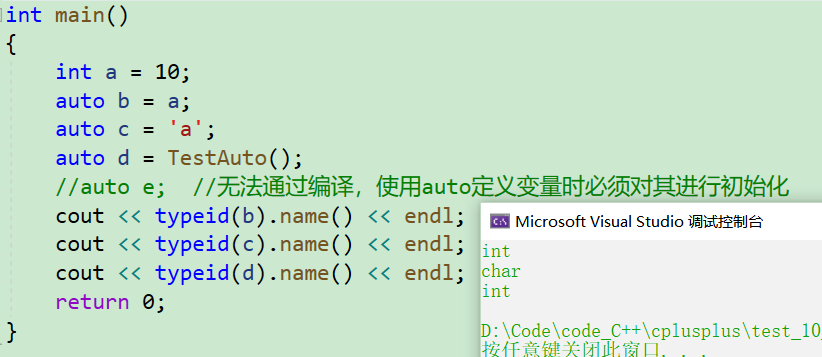• 注意：
使用auto定义变量时必须对其进行初始化，在编译阶段编译器需要根据初始化表达式来推导auto的实际类型。因此auto并非是一种“类型”的声明，而是一个类型声明时的“占位符”，编译器在编译期会将auto替换为变量实际的类型。

## 7.3.auto 的使用细则

1. auto与指针和引用结合起来使用

``````int main()
{
int x = 10;
auto a = &x;
auto* b = &x;
auto& c = x;
cout << typeid(a).name() << endl; // int*
cout << typeid(b).name() << endl; // int*
cout << typeid(c).name() << endl; // int
*a = 20;
*b = 30;
c = 40;
return 0;
}
``````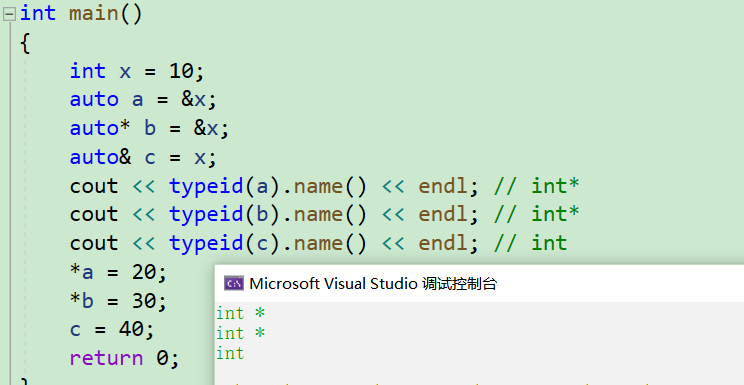1. 在同一行定义多个变量

``````void TestAuto()
{
auto a = 1, b = 2;
auto c = 3, d = 4.0; // 该行代码会编译失败，因为c和d的初始化表达式类型不同
}
``````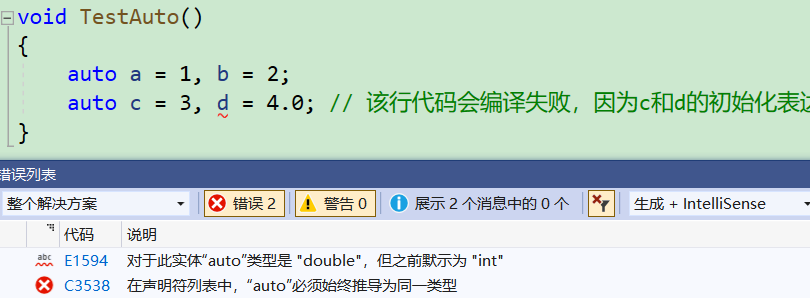## 7.4.auto不能推导的场景

1. auto不能作为函数的参数，因为不是所有的参数都有初始化表达式，因此编译器可能无法推导出a的实际类型，所以直接规定auto不能作为函数形参：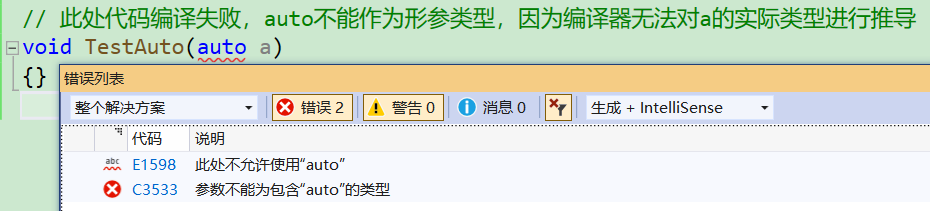1. auto不能直接用来声明数组：数组需要根据元素类型及个数来开辟空间，而数组名代表指针，因此 auto 无法推导：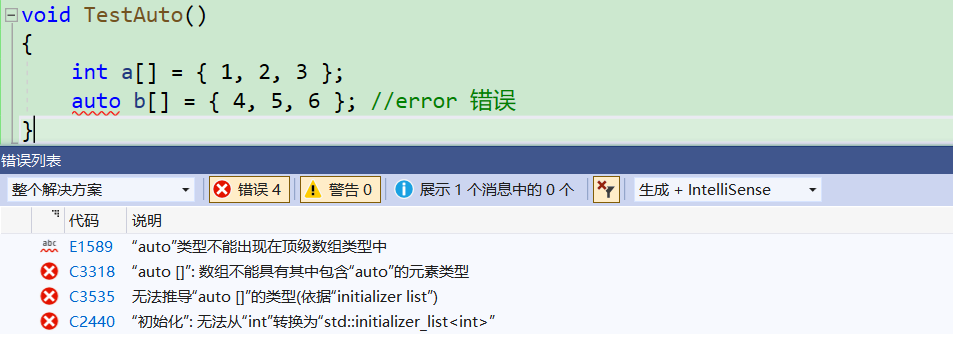1. 为了避免与C++98中的auto发生混淆，C++11只保留了auto作为类型指示符的用法；

2. auto在实际中最常见的优势用法就是跟C++11提供的新式for循环，还有lambda表达式等进行配合使用;

# 8.基于范围的 for 循环 (C++11)

## 8.1.范围 for 的用法

``````void TestFor()
{
int array[] = { 1, 2, 3, 4, 5 };
for (int i = 0; i < sizeof(array) / sizeof(array); ++i)
array[i] *= 2;
//for (int i = 0; i < sizeof(array) / sizeof(array); ++i)
for (int* p = array; p < array + sizeof(array) / sizeof(array); ++p)
cout << *p << endl;
}
``````

``````void TestFor()
{
int array[] = { 1, 2, 3, 4, 5 };
for (int i = 0; i < sizeof(array) / sizeof(int); ++i)
array[i] *= 2;
for (auto e : array)
cout << e << " "; // 2 4 6 8 10
}
``````

• 现在我们想对数组进行修改，使数组中每一个数字除以2，该怎么做呢？是如下这样嘛？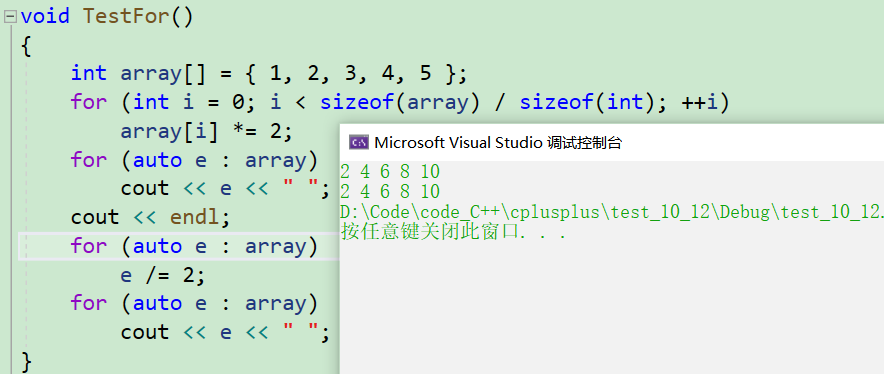``````void TestFor()
{
int array[] = { 1, 2, 3, 4, 5 };
for (int i = 0; i < sizeof(array) / sizeof(int); ++i)
array[i] *= 2;
for (auto e : array)
cout << e << " "; // 2 4 6 8 10
cout << endl;
for (auto& e : array)
e /= 2;
for (auto e : array)
cout << e << " "; //1 2 3 4 5
}
``````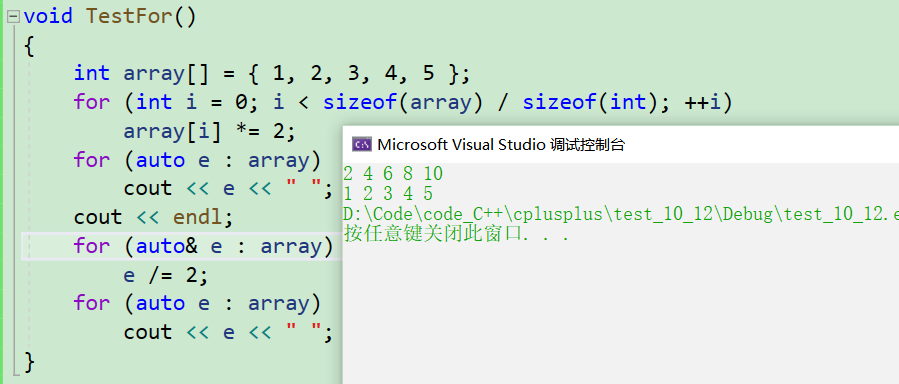1. 范围for里的auto也可以写成int，不过最好还是写成auto，毕竟auto可以自动推出数组的类型嘛，不用auto还要自己手动设置。把e改成其它的变量也是可以的，不强求。

2. 与普通循环类似，可以用continue来结束本次循环，也可以用break来跳出整个循环。

## 8.2.范围 for 的使用条件

1. for循环迭代的范围必须是确定的：对于数组而言，就是数组中第一个元素和最后一个元素的范围；对于类而言，应该提供 begin 和 end 的方法，begin 和 end 就是 for 循环迭代的范围；比如下面代码的范围就是不确定的：
``````void TestFor(int array[])
{
for(auto& e : array)
cout<< e <<endl;
}
``````

1. 迭代的对象要实现++和==的操作；(关于迭代器我们以后会学习，现在大家了解一下就可以了)

# 9.指针空值 nullptr (C++11)

``````#ifndef NULL
#ifdef __cplusplus
#define NULL   0
#else
#define NULL   ((void *)0)
#endif
#endif
``````

``````void f(int)
{
cout << "f(int)" << endl;
}

void f(int*)
{
cout << "f(int*)" << endl;
}

int main()
{
f(0);
f(NULL);
f((int*)NULL);
f(nullptr);
return 0;
}
``````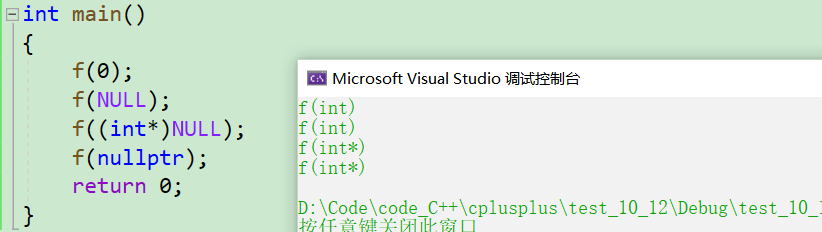nullptr 注意事项:

• 在使用nullptr表示指针空值时，不需要包含头文件，因为nullptr是C++11作为新关键字引入 的；

• 在C++11中，sizeof(nullptr) 与 sizeof((void*)0)所占的字节数相同；

• 为了提高代码的健壮性，在后续表示指针空值时建议最好使用nullptr.